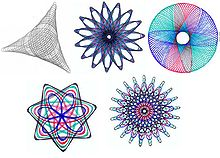Search IntMath
Close

450+ Math Lessons written by Math Professors and Teachers

5 Million+ Students Helped Each Year

1200+ Articles Written by Math Educators and Enthusiasts

Simplifying and Teaching Math for Over 23 Years

# Friday math movie: Four Cable Drawing Machine

By Murray Bourne, 10 Jan 2014

One of my favorite devices when I was a kid was my Spirograph. This allowed me to create intricate drawings which had a lovely geometry, like these:[Image source]

I came across a similar concept recently with these sand art drawing machines. This one uses 4 cables to anchor the moving object. Quite mesmerizing - and an interesting idea for a combined math-art project in school.

[Hat tip to Don Cohen for alerting me to these.]

Be the first to comment below.

### Comment Preview

HTML: You can use simple tags like <b>, <a href="...">, etc.

To enter math, you can can either:

1. Use simple calculator-like input in the following format (surround your math in backticks, or qq on tablet or phone):
a^2 = sqrt(b^2 + c^2)
(See more on ASCIIMath syntax); or
2. Use simple LaTeX in the following format. Surround your math with $$ and $$.
$$\int g dx = \sqrt{\frac{a}{b}}$$
(This is standard simple LaTeX.)

NOTE: You can mix both types of math entry in your comment.